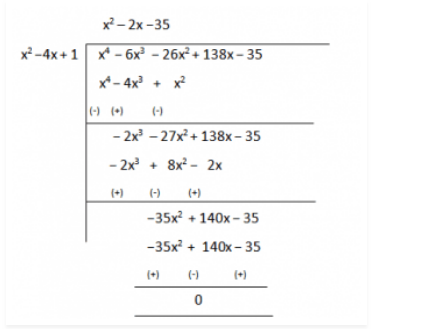Guru

# If two zeroes of the polynomial x4-6×3-26×2+138x-35 are 2 ±√3, find other zeroes. Q.4

• 0

How can i solve this question of polynomials Is it the hard question of class 10th, please suggest me the simplest way of solving this question. If two zeroes of the polynomial x4-6×3-26×2+138x-35 are 2 ±√3, find other zeroes.

Share

1. Since this is a polynomial equation of degree 4, hence there will be total 4 roots.

Let f(x) = x4-6x3-26x2+138x-35

Since 2 +√3 and 2-√3 are zeroes of given polynomial f(x).

∴ [x−(2+√3)] [x−(2-√3)] = 0

(x−2−√3)(x−2+√3) = 0

On multiplying the above equation we get,

x2-4x+1, this is a factor of a given polynomial f(x).

Now, if we will divide f(x) by g(x), the quotient will also be a factor of f(x) and the remainder will be 0.So, x4-6x3-26x2+138x-35 = (x2-4x+1)(x2 –2x−35)

Now, on further factorizing (x2–2x−35) we get,

x2–(7−5)x −35 = x2– 7x+5x+35 = 0

x(x −7)+5(x−7) = 0

(x+5)(x−7) = 0

So, its zeroes are given by:

x= −5 and x = 7.

Therefore, all four zeroes of given polynomial equation are: 2+√3 , 2-√3, −5 and 7.

• 0